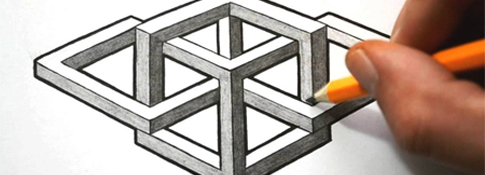##### Geometric Shapes #1Published: 3.20.2021
Level 1   |   Time: 1:54
Source: Listen In English

Improve your English with an ESL listening lesson focused on accuracy. In this lesson, you listen to a description of a series of geometric shapes. Draw the picture as you listen to the description.###Directions

1. REVIEW the vocabulary.
2. LISTEN to the audio above.
3. DRAW the picture (on a piece of paper).

###Vocabulary

If you don't know any of the words below, check the Illustrated Dictionary.

• a circle
• a square
• a triangle
• a line
• horizontal
• vertical

###Grammar (articles)

Directions: 1) Choose the determiner that you think best fits the sentence. 2) Listen again and check your answers.

• This is (a) picture of three figures – (a) triangle, (a) square and (a) circle.
• (The) triangle is on (the) left side.
• In (the) middle of (the) triangle, there is (a) vertical line.
• (The) square is in (the) middle.
• Inside (the) square, there is (a) small circle.
• (The) circle is on (the) right side.
• Inside (the) circle, there is (a) horizontal line.

###Script

This is a picture of three figures – a triangle, a square and a circle.

The triangle is on the left side.

In the middle of the triangle, there is a vertical line.

The square is in the middle.

Inside the square, there is a small circle.

The circle is on the right side.

Inside the circle, there is a horizontal line.